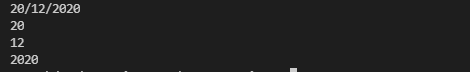Write a C++ program where you should ask the user to input a date in the format DD/MM/YYYY (like 26/01/2022) where day, month, and year are separated by a slash. Then, print day or date, month, and year on a separate line (like 26 should be printed on one line, 01 on the second line, and 2022 on the third line).

#include <iostream>
#include <string>
using namespace std;

int main(){
string date;
cin>>date;
for (int i = 0; i < date.length(); i++)
{
if (date.at(i) == '/')
{
date.erase(i, 1);
}
}
cout << date.substr(0, 2) << endl;
cout << date.substr(2, 2) << endl;
cout << date.substr(4, 7) << endl;

return 0;
}cpp programming exercise with solution